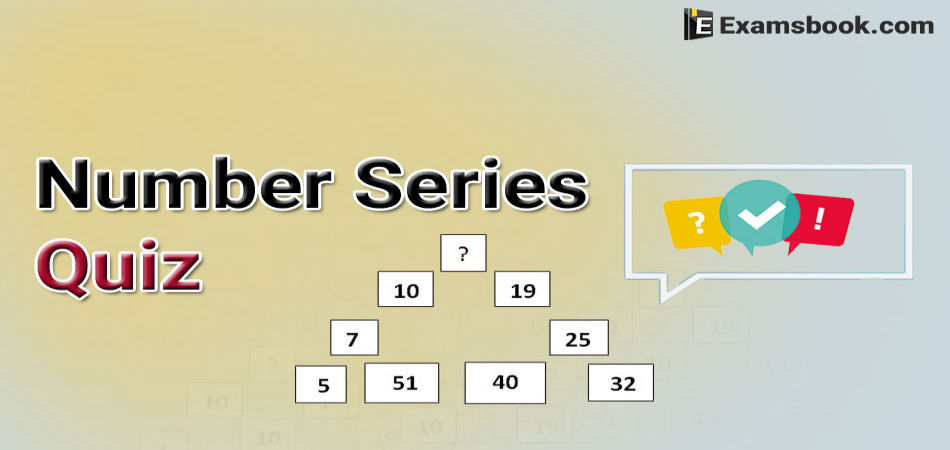• Save

# Number Series Quiz Questions for Competitive Exams

Last year 17.2K ViewsIn the number series quiz, you are given some numbers as a series and you have to find the correct number according to this series. So continue your practice by these selective number series quiz for better results in your upcoming competitive exams.

Click on Number Series Questions for more practice to improve your performance and confidence.

## Number Series Quiz for SSC and Bank Exams

Q.1. Find the missing number in the given series

2, 7, 10, 22, 18, 37, 26, ?

(A) 52

(B) 42

(C) 62

(D) 46

Ans .  A

There are two series here

2, 10, 18, 26, ... (Increase by 8)

7, 22, 37, ... (Increase by 15)

Hence, next term is 37+15 = 52

Q.2. Look at this series: 544, 509, 474, 439, ... What number should come next?

(A) 414

(B) 404

(C) 445

(D) 420

Ans .  B

This is a simple subtraction series. Each number is 35 less than the previous number.

Q.3. Find the missing number in the given series

279936, 46656, 7776, 1296, 216, ?

(A) 46

(B) 60

(C) 66

(D) 36

Ans .  D

Go on dividing by 6 to the next number

Q.4. Look at this series: 201, 202, 204, 207, ... What number should come next?

(A) 208

(B) 205

(C) 211

(D) 210

Ans .  C

In this addition series, 1 is added to the first number; 2 is added to the second number; 3 is added to the third number; 4 is added to the fourth number; and go on.

Q.5. Find the missing number in the given series

12, 38, 116, 350, 1052, ?

(A) 2200

(B) 1800

(C) 3158

(D) 2800

Ans .  C

12 × 3 + 2 = 38

38 × 3 + 2 = 116

116 × 3 + 2 = 350

350 × 3 + 2 = 1052

1052 × 3 + 2 = 3158

Q.6. Look at this series: 2, 4, 6, 8, 10, ... What number should come next?

(A) 12

(B) 11

(C) 14

(D) 13

Ans .  A

This is a simple addition series. Each number increases by 2.

Q.7. Find the missing number in the given series

46080, 3840, 384, 48, 8, 2, ?

(A) 1/64

(B) 1

(C) 2

(D) 1/8

Ans .  B

46080 /12 = 3840

3840 /10 = 384

384 /8 = 48

48 /6 = 8

8/4 = 2

2 /2 = 1

Q.8. Look at this series: 14, 28, 20, 40, 32, 64, ... What number should come next?

(A) 56

(B) 52

(C) 128

(D) 96

Ans .  A

This is an alternating multiplication and subtracting series: First, multiply by 2 and then subtract 8.

Q.9. Find the missing number in the given series

5,28,57,88,125

(A) 147

(B) 156

(C) 186

(D) 166

Ans .  D

28=23+5

57=29+28

88=31+57

125=37+88

166=41+125

Q.10. Look at this series: 1.5, 2.3, 3.1, 3.9, ... What number should come next?

(A) 4.4

(B) 4.2

(C) 5.1

(D) 4.7

Ans .  D

In this simple addition series, each number increases by 0.8.

If you are facing any problem regarding the number series quiz, ask me in the comment section without any hesitation. Visit on the next page for more practice.